GeeksforGeeks App
Open AppBrowser
Continue

# Compute element-wise logical AND, OR and NOT of tensors in PyTorch

In this article, we are going to see how to compute element-wise logical AND, OR, and NOT of given tensors in PyTorch. We can compute this by using the torch.logical_and(), torch.logical_or(), and torch.logical_not() methods. Let’s discuss all of them one by one.

## Compute element-wise with logical AND

torch.logical_and() – This method is used to compute the element-wise logical AND of the given tensor. This method treated the non-zero values as True and zero values as False. The following syntax is used to compute logical AND.

Syntax: torch.logical_and(input, other)

Parameters

• input –  This is our input tensor
• other – This tensor is to compute AND with input tensor.

Return : This method returns a tensor with values we get after computing the logical AND.

Example 1:

The following program is to compute element-wise logical AND on two 1D tensors having boolean values.

## Python3

 `# Import the required library``import` `torch`` ` `# create two tensors having boolean values``tens_1 ``=` `torch.tensor([``True``, ``True``, ``False``, ``False``])``tens_2 ``=` `torch.tensor([``True``, ``False``, ``True``, ``False``])`` ` `# display the above created tensors``print``(``"Input Tensor 1: "``, tens_1)``print``(``"Input Tensor 2: "``, tens_2)`` ` `# compute the logical AND of input1 and input2``tens ``=` `torch.logical_and(tens_1, tens_2)`` ` `# print result``print``(``"\nAfter Compute Logical AND: "``, tens)`

Output: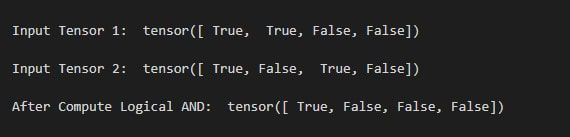Example 2:

The following program is to understand how to compute element-wise logical AND on two 2D tensors.

## Python3

 `# Import the required library``import` `torch`` ` `# create two tensors``tens_1 ``=` `torch.tensor([[``10``, ``0``], [``0``, ``20``]])``tens_2 ``=` `torch.tensor([[``0``, ``30``], [``0``, ``40``]])`` ` `# display the tensors``print``(``"Input Tensor 1: \n"``, tens_1)``print``(``"Input Tensor 2: \n"``, tens_2)`` ` `# compute the logical AND``tens ``=` `torch.logical_and(tens_1, tens_2)`` ` `# print result``print``(``"After Compute Logical AND: \n"``, tens)`

Output: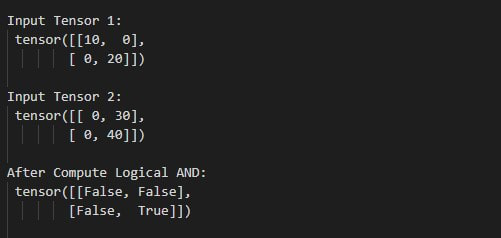## Compute element-wise with logical OR

torch.logical_or() – This method is used to compute the element-wise logical OR of the given tensor. This method also treated the non-zero values as True and zero values as False. The following syntax is used to compute logical OR.

Syntax: torch.logical_or(input, other)

Parameters

• input –  This is our input tensor
• other – This tensor is to compute OR with input tensor.

Return –returns a tensor with values we get after computing the logical OR.

Example 1:

The following program is to know how to compute element-wise logical OR on two 1D tensors.

## Python3

 `# Import the required library``import` `torch`` ` `# create two tensors``tens_1 ``=` `torch.tensor([[``10``, ``0``, ``20``, ``0``]])``tens_2 ``=` `torch.tensor([[``0``, ``30``, ``40``, ``0``]])`` ` `# display the tensors``print``(``"\n Input Tensor 1: "``, tens_1)``print``(``"\n Input Tensor 2: "``, tens_2)`` ` `# compute the logical OR``tens ``=` `torch.logical_or(tens_1, tens_2)`` ` `# print result``print``(``"\n After Compute Logical OR: "``, tens)`

Output: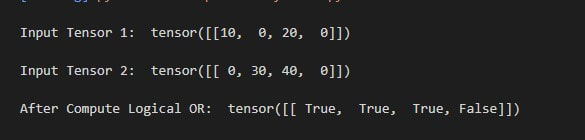Example 2:

The following program is to understand how to compute element-wise logical OR on two 2D tensors.

## Python3

 `# Import the required library``import` `torch`` ` `# create two tensors``tens_1 ``=` `torch.tensor([[``11``, ``0``], [``0``, ``12``]])``tens_2 ``=` `torch.tensor([[``0``, ``13``], [``0``, ``14``]])`` ` `# display the tensors``print``(``"\n Input Tensor 1: \n"``, tens_1)``print``(``"\n Input Tensor 2: \n"``, tens_2)`` ` `# compute the logical OR``tens ``=` `torch.logical_or(tens_1, tens_2)`` ` `# print result``print``(``"\n After Compute Logical OR: \n"``, tens)`

Output: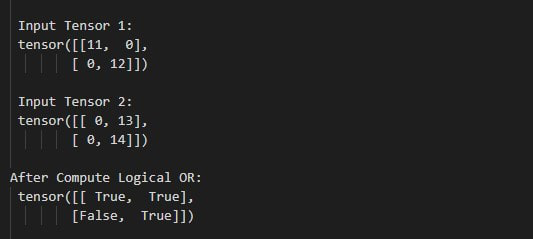## Compute element-wise with logical NOT

torch.logical_not() – This method is used to compute the element-wise logical NOT of the given input tensor. This method also treated the non-zero values as True and zero values as False.  The following syntax is used to compute logical NOT.

Syntax – torch.logical_not(input)

Parameter –

• input – This is our input tensor

Return –This method returns a tensor with values we get after computing the logical NOT.

The following program is to understand how to compute element-wise logical NOT of tensor.

## Python3

 `# import required library``import` `torch`` ` `# create two tensors``tens_1 ``=` `torch.tensor([``11``, ``0``])`` ` ` ` `# display the tensors``print``(``"\n Input Tensor 1: \n"``, tens_1)`` ` `# compute the logical NOT``tens ``=` `torch.logical_not(tens_1)`` ` `# display result``print``(``"\n After Compute Logical NOT: \n"``, tens)`

Output: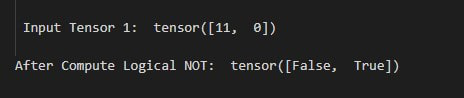My Personal Notes arrow_drop_up